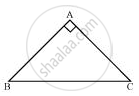# ABC is a right angled triangle in which ∠A = 90º and AB = AC. Find ∠B and ∠C. - Mathematics

ABC is a right angled triangle in which ∠A = 90º and AB = AC. Find ∠B and ∠C.

#### SolutionIt is given that

AB = AC

⇒ ∠C = ∠B (Angles opposite to equal sides are also equal)

In ΔABC,

∠A + ∠B + ∠C = 180º (Angle sum property of a triangle)

⇒ 90º + ∠B + ∠C = 180º

⇒ 90º + ∠B + ∠B = 180º

⇒ 2 ∠B = 90º

⇒ ∠B = 45º

∴ ∠B = ∠C = 45º

Concept: Properties of a Triangle
Is there an error in this question or solution?

#### APPEARS IN

NCERT Class 9 Maths
Chapter 7 Triangles
Exercise 7.2 | Q 7 | Page 124

Share